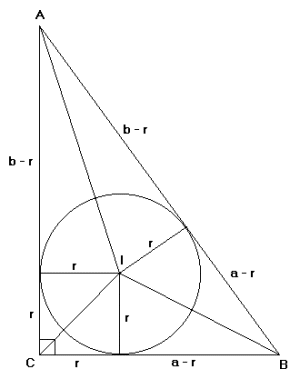The Inradius of a Right Triangle With Integral Sides
Bill Richardson
September 1999

Let a = x2 - y2, b = 2xy, c = x2 + y2 with 0 < y < x, (x,y) = 1 and x and y being of opposite parity. Then (a, b, c) is a primative Pythagorean triple. Let triangle ABC, in the figure below, be a right triangle with sides a, b and hypotenuse c. Let the circle with center I be the inscribed circle for this triangle. We will prove that the inradius, r, is an integer.Proof. Let r be the inradius. Since the tangents to a circle from a point outside the circle are equal, we have the sides of triangle ABC configured as in the above figure. Thus, c = (a - r) + (b - r) = a + b - 2r and r = (a + b - c)/2. But a = x2 - y2, b = 2xy, c = x2 + y2 which gives r = (x2 - y2 + 2xy - x2 - y2)/2 = (2xy - 2y2)/2 = y(x - y). Thus, r is an integer given by r = y(x - y).

An alternate proof uses the fact that

AreaTriangleABC = AreaTriangleAIB + AreaTriangleBIC + AreaTriangleAIC.

So, (½)ab = (½)rc + (½)ra + (½)rb and
r = ab/(a + b + c) = (x2 - y2)2xy/(x2 - y2 + 2xy + x2 + y2) = [2xy(x - y)(x + y)]/[2x(x + y)] = y(x - y).
Thus, r is an integer given by r = y(x - y).